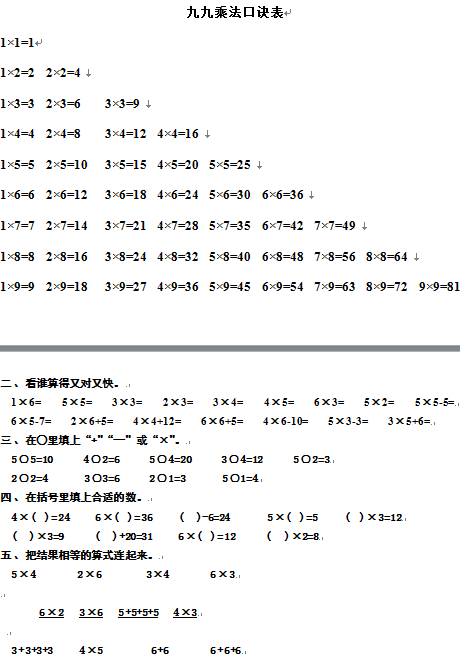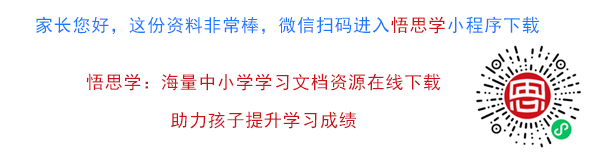1×1=1

1×2=2  2×2=4

1×3=3  2×3=6    3×3=9

【DOC文档6页】小学二年级数学上册乘法口诀表+测试卷 A4电子版资料_可直接打印_会员免费下载##### 评论信息